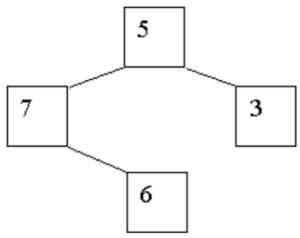TORONTO KIDS COMPUTER CLUB | PMCA Sunday 10:00 Practice -10.17
19967

# PMCA Sunday 10:00 Practice -10.17

## 14 Oct PMCA Sunday 10:00 Practice -10.17

Question:
Each year, fall in the North Central region is accompanied by the brilliant colors of the leaves on the trees, followed quickly by the falling leaves accumulating under the trees. If the same thing happened to binary trees, how large would the piles of leaves become?

We assume each node in a binary tree ”drops” a number of leaves equal to the integer value stored in that node. We also assume that these leaves drop vertically to the ground (thankfully, there’s no wind to blow them around). Finally, we assume that the nodes are positioned horizontally in such a manner that the left and right children of a node are exactly one unit to the left and one unit to the right, respectively, of their parent. Consider the following tree on the right:The nodes containing 5 and 6 have the same horizontal position (with different vertical positions, of course). The node containing 7 is one unit to the left of those containing 5 and 6, and the node containing 3 is one unit to their right. When the ”leaves” drop from these nodes, three piles are created: the leftmost one contains 7 leaves (from the leftmost node), the next contains 11 (from the nodes containing 5 and 6), and the rightmost pile contains 3. (While it is true that only leaf nodes in a tree would logically have leaves, we ignore that in this problem.)

Input Specification:
The input contains multiple test cases, each describing a single tree. A tree is specified by giving the value in the root node, followed by the description of the left subtree, and then the description of the right subtree. If a subtree is empty, the value ‘-1’ is supplied. Thus the tree shown above is specified as ‘5 7 -1 6 -1 -1 3 -1 -1’. Each actual tree node contains a positive, non-zero value. The last test case is followed by a single ‘-1’ (which would otherwise represent an empty tree).

Output Specification:
For each test case, display the case number (they are numbered sequentially, starting with 1) on a line by itself. On the next line display the number of “leaves” in each pile, from left to right, with a single space separating each value. This display must start in column 1, and will not exceed the width of an 80-character line. Follow the output for each case by a blank line. This format is illustrated in the examples below.

```Sample Input
5 7 -1 6 -1 -1 3 -1 -1
8 2 9 -1 -1 6 5 -1 -1 12 -1 -1 3 7 -1 -1 -1
-1

Sample Output
Case 1:
7 11 3

Case 2:
9 7 21 15```